This site uses cookies. By continuing to browse the ConceptDraw site you are agreeing to our Use of Site Cookies.
ConceptDraw Samples | Engineering Diagrams

# Engineering

Samples of technical drawings and engineering diagrams (electrical schematics, digital and analog logic, circuit and wiring schematics and diagrams; power systems diagrams; maintenance and repair diagrams for electronics and electrical engineering; block flow and process flow diagrams, piping and instrumentation diagrams for chemical and process engineering; part, assembly, pneumatic, and hydraulic systems drawings for mechanical engineering) are created using ConceptDraw DIAGRAM diagramming and vector drawing software enhanced with Engineering solution from ConceptDraw Solution Park. ConceptDraw DIAGRAM provides export of vector graphic multipage documents into multiple file formats: vector graphics (SVG, EMF, EPS), bitmap graphics (PNG, JPEG, GIF, BMP, TIFF), web documents (HTML, PDF), PowerPoint presentations (PPT), Adobe Flash (SWF).

## Tutorials and Solutions:

Video Tutorials: ConceptDraw Solution Park

Solutions: Engineering for ConceptDraw DIAGRAM

## Sample 1: Process Flow Diagram (PFD)

Process Flow Diagram (PFD) sample: Industrial Design.

This example is created using ConceptDraw DIAGRAM
diagramming and vector drawing software enhanced with
Engineering solution from ConceptDraw Solution Park.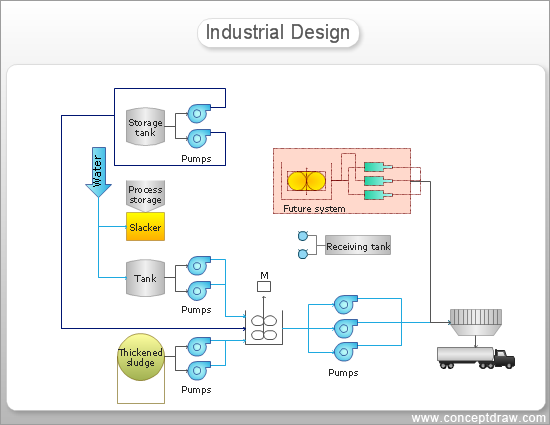## Sample 2: Process Flow Diagram (PFD)

Process Flow Diagram (PFD) sample: Drinking Water System.

This example is created using ConceptDraw DIAGRAM
diagramming and vector drawing software enhanced with
Engineering solution from ConceptDraw Solution Park.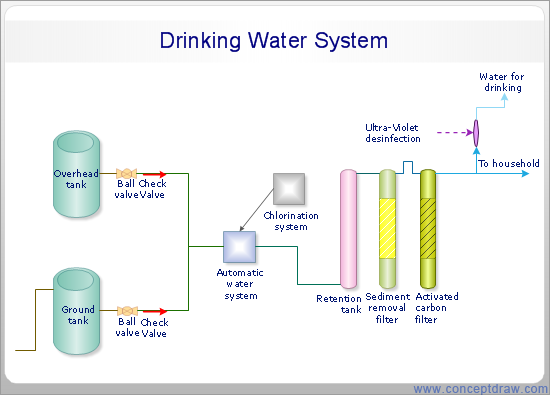## Sample 3: Piping and Instrumentation Diagram (P&ID)

Piping and Instrumentation Diagram (P&ID) sample: Diagram of magnetite pulp reclaiming.

This example is created using ConceptDraw DIAGRAM
diagramming and vector drawing software enhanced with
Engineering solution from ConceptDraw Solution Park.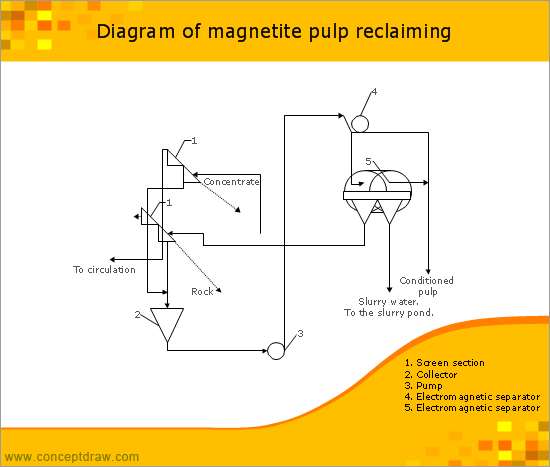## Sample 4: Electrical Diagram

Electrical Diagram sample.

This example is created using ConceptDraw DIAGRAM
diagramming and vector drawing software enhanced with
Engineering solution from ConceptDraw Solution Park.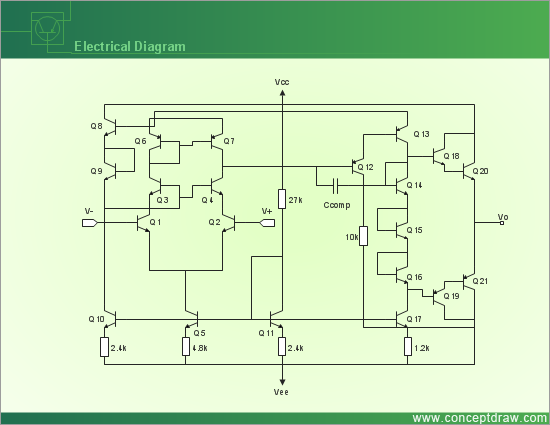## Sample 5: Electrical Circuit Diagram

Electrical Circuit Diagram sample: Personal Pocket Pager Circuit Diagram.

This example is created using ConceptDraw DIAGRAM
diagramming and vector drawing software enhanced with
Engineering solution from ConceptDraw Solution Park.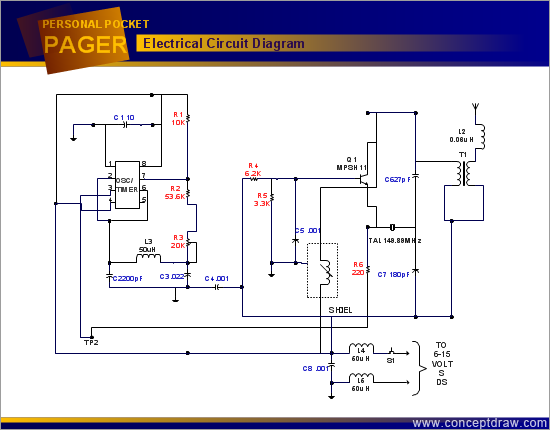## Sample 6: Technical Drawing — Die Stacking

Technical Drawing sample: Die stacking.

This example is created using ConceptDraw DIAGRAM
diagramming and vector drawing software enhanced with
Engineering solution from ConceptDraw Solution Park.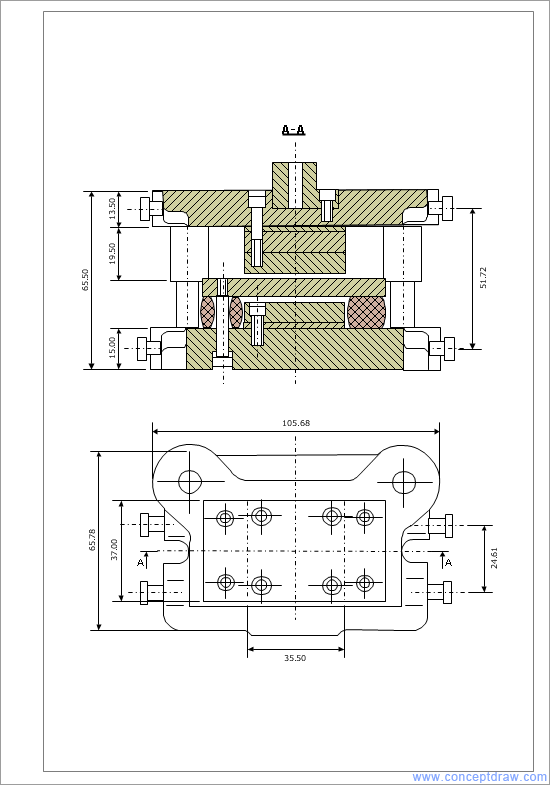## Sample 7: Root Cause Analysis Tree Diagram — Manufacturing Problem Solution

Root Cause Analysis Tree Diagram sample — Manufacturing problem solution.

This example is created using ConceptDraw DIAGRAM diagramming and vector drawing software enhanced with Engineering solution from ConceptDraw Solution Park.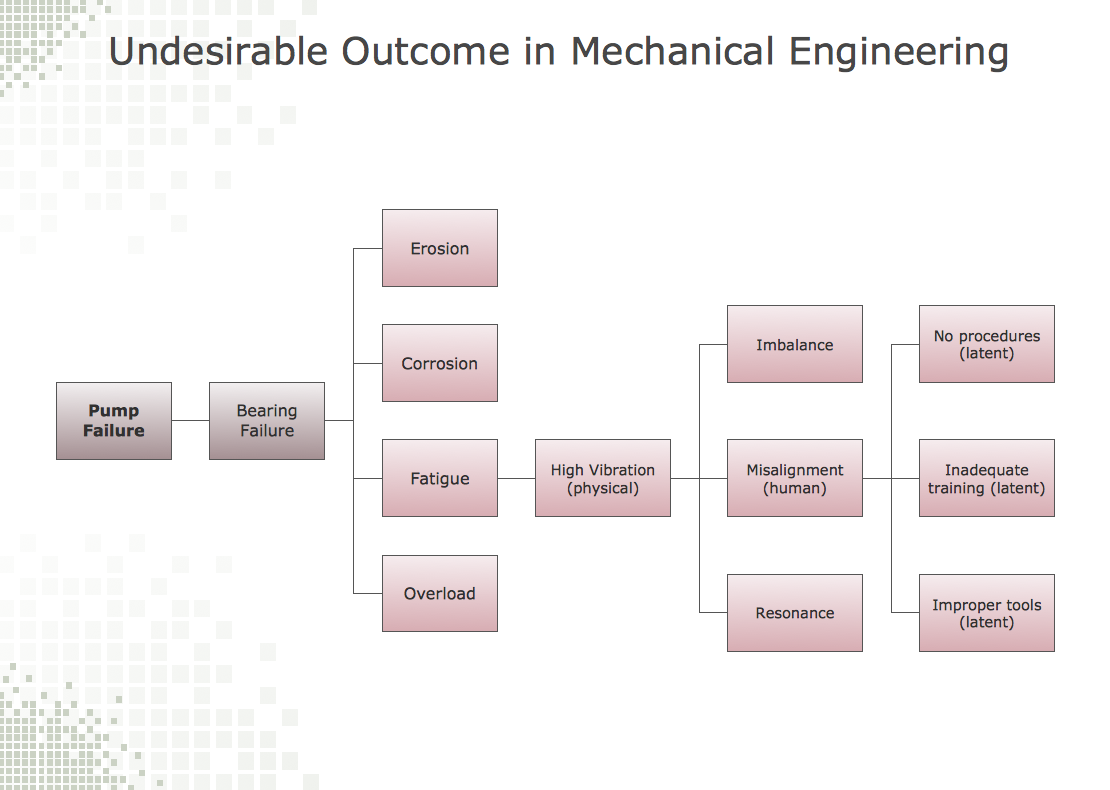## Sample 8: Process Flow Diagram (PFD) — Drinking Water System

Process Flow Diagram (PFD) sample: Drinking water system.

This example is created using ConceptDraw DIAGRAM diagramming and vector drawing software enhanced with Engineering solution from ConceptDraw Solution Park.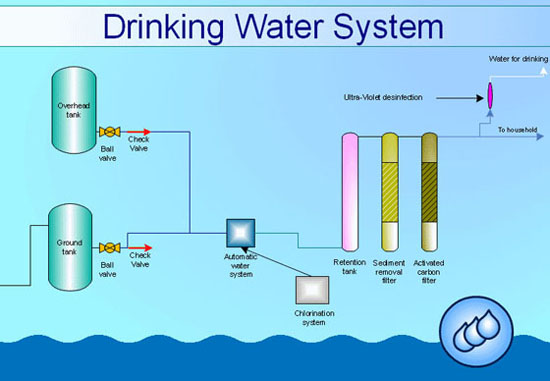## Sample 9: Process Flow Diagram (PFD) — Industrial Design

Process Flow Diagram (PFD) sample: Industrial design.

This example is created using ConceptDraw DIAGRAM diagramming and vector drawing software enhanced with Engineering solution from ConceptDraw Solution Park.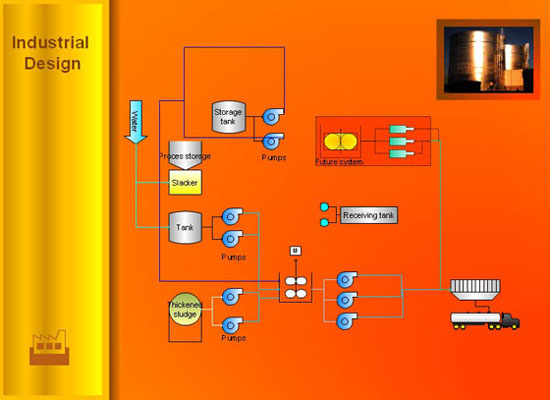All samples are copyrighted CS Odessa's.

Usage of them is covered by Creative Commons “Attribution Non-Commercial No Derivatives” License.

The text you can find at: https://creativecommons.org/licenses/by-nc-nd/3.0